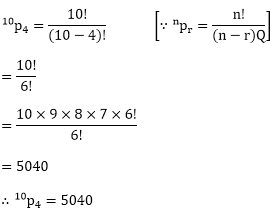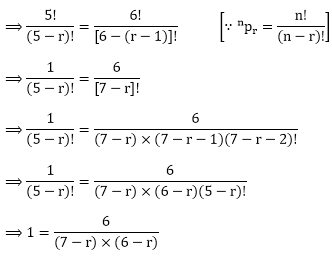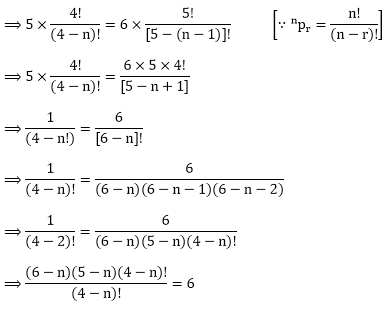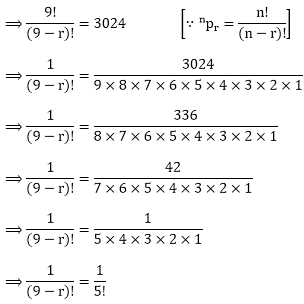×#### Thank you for registering.

One of our academic counsellors will contact you within 1 working day.

Click to Chat

1800-1023-196

+91-120-4616500

CART 0

• 0

MY CART (5)

Use Coupon: CART20 and get 20% off on all online Study Material

ITEM
DETAILS
MRP
DISCOUNT
FINAL PRICE
Total Price: Rs.

There are no items in this cart.
Continue Shopping• Complete JEE Main/Advanced Course and Test Series
• OFFERED PRICE: Rs. 15,900
• View Details

```Chapter 16: Permutations – Exercise 16.3

Permutations – Exercise – 16.3 – Q.1(i)

We have,Permutations – Exercise – 16.3 – Q.1(ii)

We have,Permutations – Exercise – 16.3 – Q.1(iii)

We have,Permutations – Exercise – 16.3 – Q.1(iv)

We have,= 360

Hence, p(6, 4) = 360

Permutations – Exercise – 16.3 – Q.2

We have,

p(5, r) = p(6, r - 1)⟹ (6 - r) × (7 - r) = 6

⟹ 42 - 6r - 7r + r2 = 6

⟹ r2- 12r + 42 – 6 = 0

⟹ r2 - 13r + 36 = 0

⟹r2 - 9r - 4r + 36 = 0

⟹r(r - 9) - 4(r - 9) = 0

⟹ (r - 9)(r - 4) = 0Hence, r = 4

Permutations – Exercise – 16.3 – Q.3

We have,

5p(4, n) = 6, p(5, n - 1)⟹ (6 - n)(5 - n) = 6

⟹ 30 - 6n - 5n + n2 = 6

⟹ n2- 11n + 24 = 0

⟹ n2- 8n - 3n + 24 = 0

⟹ n(n - 8) - 3(n - 8) = 0

⟹ (n - 8)(n - 3) = 0⟹ n = 3

Hence, n = 3

Permutations – Exercise – 16.3 – Q.4

We have

p(n, 5) = 20, p(n, 3)⟹ (n - 3)(n - 4) = 20

⟹ n2- 4n - 3n + 12 = 20

⟹ n2- 7n – 8 = 0

⟹n(n - 8) + 1(n - 8) = 0

⟹ (n - 8)(n + 1) = 0

⟹ n – 8 = 0             [∵ n ≠ - 1]

⟹ n = 8

Hence, n = 8

Permutations – Exercise – 16.3 – Q.5

We have,⟹ n(n - 1)(n - 2)(n - 3) = 6 × 5 × 4 × 3

⟹ n = 6         [13y comparing]

Hence, n = 6

Permutations – Exercise – 16.3 – Q.6

We have,

p(9, r) =  3024⟹ (9 - r)! = 5!

⟹ 9 – r = 5

⟹ 9 – 5 = r

⟹ 4 = r

⟹ r = 4

Hence, r = 4

```### Course Features

• 728 Video Lectures
• Revision Notes
• Previous Year Papers
• Mind Map
• Study Planner
• NCERT Solutions
• Discussion Forum
• Test paper with Video Solution